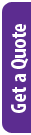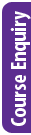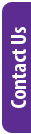## Details

Python has a fantastic array of modules that are useful to data scientists. Easily enabling data processing, modelling, linear algebra and much more. Python has rich data visualization capabilities, fast numerical methods, statistical and data analysis tools and machine learning libraries.

In this course you will learn how to:

• Perform complex maths in Python
• Solve mathematical equations symbolically using python
• Use numerical methods to find solutions to mathematical problems
• Analyze data
• Create amazing visualizations
• Interact with Power BI
• Introduce machine learning

### Delivery Methods

Delivery Method Duration Price (excl. VAT)
Fulltime 2 Days R 5,500.00
Webinar 2 Days R 4,600.00

### Discounts Available

Save up to 10% by booking and paying 10 business days before the course.

### Brochure:

Information may change without notice.

## Audience

Would be data scientists, scientists, engineers, data analysts.

## Pre-Requisites

Introduction to programming using Python or equivalent knowledge.  See https://www.leadingtraining.co.za/introduction_to_programming_training_course

## Course Outline / Curriculum

### Symbolic Math (symy)

Symbols

isympy

Numeric types

Differentiation and Integration

Ordinary differential equations

Series expansions and plotting

Linear equations and matrix inversion

Non linear equations

Output: LATEX interface and pretty-printing

### Numerical Python (numpy)

Introduction

Arrays (Matrices)

Convert from array to list or tuple

Standard Linear Algebra operations

Numpy for Matlab users

### Visualising Data

Matplotlib 2D plotting

Matplotlib and Pylab

pyplot, numpy

Plotting more than one curve

Histograms

Visualising matrix data

3D Plots

### Numerical Methods (scipy)

Overview

Numerical integration

Solving ordinary differential equations

Finding roots using the bisection and fsolve method

Fast Fourier Transforms

Interpolation and curve fitting, including Linear regression

Intro to Machine Learning

Predictive models

Supervised Learning, Naive Bayes, Decision Trees, Random Forests.

Unsupervised Learning

## Schedule Dates and Booking

To apply for a booking, click on the relevant "Book Now" button below.
Note: places are only fully secured once payment has been made.

Start Date Branch